## Example Questions

### Example Question #59 : Trigonometry

Find the domain of the function: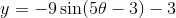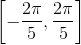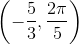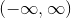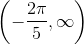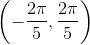Explanation:

The functionis related to the parent function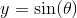, which has a domain of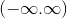.

The value of theta forhas no restriction and is valid for all real numbers.

The answer is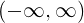.

### Example Question #60 : Trigonometry

What is the domain of the given trigonometric function: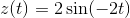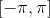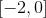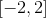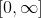For both Sine and Cosine, since there are no asymptotes like Tangent and Cotangent functions, the function can take in any value for. Thus the domain is: